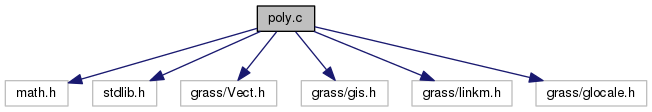GRASS Programmer's Manual  6.5.svn(2014)-r66266
Vlib/poly.c File Reference
`#include <math.h>`
`#include <stdlib.h>`
`#include <grass/Vect.h>`
`#include <grass/gis.h>`
`#include <grass/linkm.h>`
`#include <grass/glocale.h>`
Include dependency graph for Vlib/poly.c:Go to the source code of this file.

struct  Slink

## Functions

int Vect__intersect_line_with_poly ()

int Vect_get_point_in_area (struct Map_info *Map, int area, double *X, double *Y)
Get point inside area and outside all islands. More...

int Vect__intersect_line_with_poly (struct line_pnts *Points, double y, struct line_pnts *Inter)

int Vect_get_point_in_poly (struct line_pnts *Points, double *X, double *Y)
Get point inside polygon. More...

int Vect_find_poly_centroid (struct line_pnts *points, double *cent_x, double *cent_y)
Get centroid of polygon. More...

int Vect_get_point_in_poly_isl (struct line_pnts *Points, struct line_pnts **IPoints, int n_isles, double *att_x, double *att_y)
Get point inside polygon but outside the islands specifiled in IPoints. More...

int Vect_point_in_poly (double X, double Y, struct line_pnts *Points)
Determines if a point (X,Y) is inside a polygon. More...

int Vect_point_in_area_outer_ring (double X, double Y, struct Map_info *Map, int area)
Determines if a point (X,Y) is inside an area outer ring. Islands are not considered. More...

int Vect_point_in_island (double X, double Y, struct Map_info *Map, int isle)
Determines if a point (X,Y) is inside an island. More...

## Function Documentation

 int Vect__intersect_line_with_poly ( )
 int Vect__intersect_line_with_poly ( struct line_pnts * Points, double y, struct line_pnts * Inter )

Definition at line 141 of file Vlib/poly.c.

References b, and Vect_append_point().

 int Vect_find_poly_centroid ( struct line_pnts * points, double * cent_x, double * cent_y )

Get centroid of polygon.

Parameters
 points polygon [out] cent_x,cent_y centroid coordinates
Returns
0 on success
-1 on error

Definition at line 330 of file Vlib/poly.c.

Referenced by Vect_get_point_in_poly(), and Vect_get_point_in_poly_isl().

 int Vect_get_point_in_area ( struct Map_info * Map, int area, double * X, double * Y )

Get point inside area and outside all islands.

Take a line and intersect it with the polygon and any islands. sort the list of X values from these intersections. This will be a list of segments alternating IN/OUT/IN/OUT of the polygon. Pick the largest IN segment and take the midpoint.

Parameters
 Map vector map area area id [out] X,Y point coordinateds
Returns
0 on success
-1 on error

Definition at line 61 of file Vlib/poly.c.

Referenced by wxdigit.IVDigit::InitCats().

 int Vect_get_point_in_poly ( struct line_pnts * Points, double * X, double * Y )

Get point inside polygon.

This does NOT consider ISLANDS!

Parameters
 Points polygon [out] X,Y point coordinates
Returns
0 on success
-1 on error

Definition at line 180 of file Vlib/poly.c.

 int Vect_get_point_in_poly_isl ( struct line_pnts * Points, struct line_pnts ** IPoints, int n_isles, double * att_x, double * att_y )

Get point inside polygon but outside the islands specifiled in IPoints.

Take a line and intersect it with the polygon and any islands. sort the list of X values from these intersections. This will be a list of segments alternating IN/OUT/IN/OUt of the polygon. Pick the largest IN segment and take the midpoint.

Parameters
 Points polygon IPoints isles n_isles number of isles [out] att_x,att_y point coordinates
Returns
0 on success
-1 on error

Definition at line 424 of file Vlib/poly.c.

Referenced by Vect_get_point_in_area().

 int Vect_point_in_area_outer_ring ( double X, double Y, struct Map_info * Map, int area )

Determines if a point (X,Y) is inside an area outer ring. Islands are not considered.

Parameters
 X,Y point coordinates Map vector map area area id
Returns
0 - outside
1 - inside
2 - on the boundary (exactly may be said only for vertex of vertical/horizontal line)

Definition at line 695 of file Vlib/poly.c.

References first, G_debug(), NULL, Vect_new_line_struct(), and Vect_read_line().

Referenced by Vect_isle_find_area(), and Vect_point_in_area().

 int Vect_point_in_island ( double X, double Y, struct Map_info * Map, int isle )

Determines if a point (X,Y) is inside an island.

Parameters
 X,Y point coordinates Map vector map isle isle id
Returns
0 - outside
1 - inside
2 - on the boundary (exactly may be said only for vertex of vertical/horizontal line)

Definition at line 760 of file Vlib/poly.c.

References first, G_debug(), NULL, Vect_new_line_struct(), and Vect_read_line().

Referenced by Vect_find_island(), and Vect_point_in_area().

 int Vect_point_in_poly ( double X, double Y, struct line_pnts * Points )

Determines if a point (X,Y) is inside a polygon.

Parameters
 X,Y point coordinates Points polygon
Returns
0 - outside
1 - inside
2 - on the boundary (exactly may be said only for vertex of vertical/horizontal line)

Definition at line 665 of file Vlib/poly.c.

References G_debug().